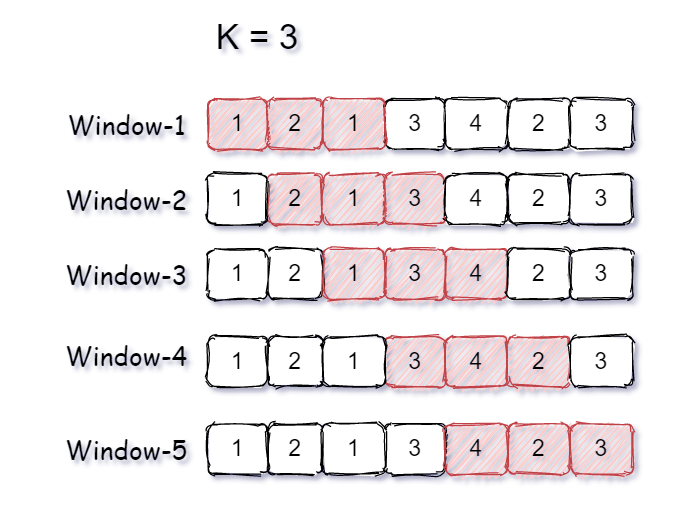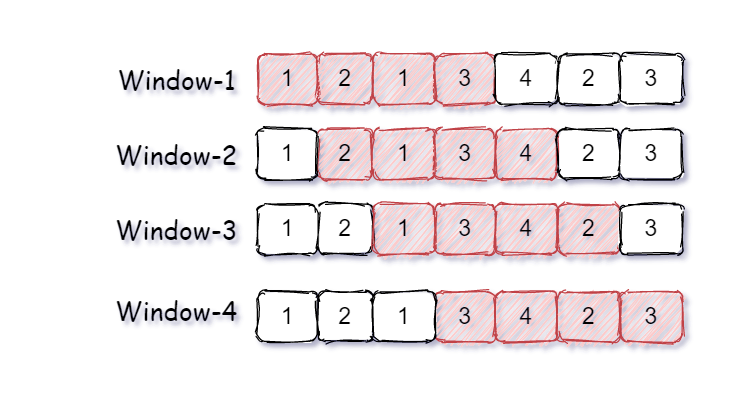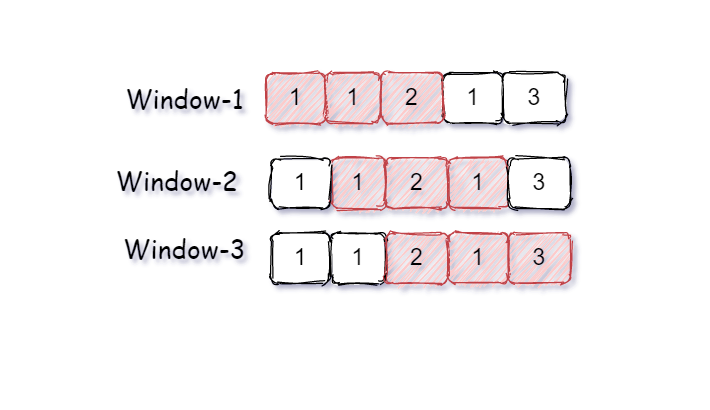13

Count Distinct Element in Every K Size Window

Difficulty: EASY
Avg. time to solve
15 min
Success Rate
85%

Problem Statement

You are given an array ‘ARR’ of size ‘N’ and an integer ‘K’. Your task is to find the total number of distinct elements present in every ‘K’ sized window of the array. A ‘K’ sized window can also be viewed as a series of continuous ‘K’ elements present in the sequence.

Note:
1. The size of ‘ARR’ will always be greater than or equal to the ‘K’.
2. Here window refers to a subarray of ‘ARR’. Hence ‘K’ sized window means a subarray of size ‘K’.
3. You are not required to print the output explicitly. It has already been taken care of. Just implement the function and return an array of the count of all distinct elements in the ‘K’ size window.

Example

Consider ARR = [ 1, 2, 1, 3, 4, 2, 3 ] and K = 3.As per the given input, we have a sequence of numbers of length 7, and we need to find the number of distinct elements present in all the windows of size 3.

Window-1 has three elements { 1, 2, 1 } and only two elements { 1, 2 } are distinct because 1 is repeating two times.
Window-2 has three elements { 2, 1, 3 } and all three elements are distinct { 2, 1, 3 }.
Window-3 has three elements { 1, 3, 4 } and all three elements are distinct { 1, 3, 4 }.
Window-4 has three elements { 3, 4, 2 } and all three elements are distinct { 3, 4, 2 }.
Window-5 has three elements { 4, 2, 3 } and all three elements are distinct { 4, 2, 3 }.

Hence, the count of distinct elements in all K sized windows is { 2, 3, 3, 3, 3 }.
Input format:
The first line of the input contains an integer, 'T,’ denoting the number of test cases.
The first line of each test case contains two space-separated integers, 'N' and ‘K’, denoting the number of elements in the array and the size of the window.
The second line of each test case contains 'N' space-separated integers denoting the elements of the array 'ARR'.
Output Format:
For each test case, print an array that contains the number of distinct elements in all ‘K’ size windows, and the count of distinct elements must be from the left to the right window.

Print the output of each test case in a separate line.
Constraints:
1 <= T <= 10
1 <= N <= 10 ^ 5
1 <= K <= N
1 <=ARR[i] <= 10 ^ 9

Where 'T' denotes the number of test cases, 'N' denotes the number of elements in the array, ‘K’ denotes the size of the window, and 'ARR[i]' denotes the 'i-th' element of the array 'ARR'.

Time limit: 1 second
2
7 4
1 2 1 3 4 2 3
5 3
1 1 2 1 3
3 4 4 3
2 2 3
Explanation of sample input 1:
Test Case 1:Window-1 has four elements { 1, 2, 1, 3 } and only three elements { 1, 2, 3 } are distinct because 1 is repeating two times.
Window-2 has four elements { 2, 1, 3, 4 } and all four elements { 2, 1, 3, 4 } are distinct.
Window-3 has four element { 1, 3, 4, 2 } and all four elements { 1, 3, 4, 2 } are distinct.
Window-4 has four element { 3, 4, 2, 3 } and only three elements { 3, 4, 2 } are distinct because 3 is repeating two times.

Hence, the count of distinct elements in all windows is { 3, 4, 4, 3}.

Test case 2:Window-1 has three elements { 1, 1, 2 } and only two elements { 1, 2 } are distinct because 1 is repeating two times.
Window-2 has three elements { 1, 2, 1 } and only two elements { 2, 1 } are distinct.
Window-3 has three elements { 2, 1, 3 } and all three elements { 2, 1, 3 } are distinct.

Hence, the count of distinct elements in all windows is { 2, 2, 3 }.
2
4 1
2 3 1 2
5 2
2 2 3 2 1
Sample Output 2:
1 1 1 1
1 2 2 2Console